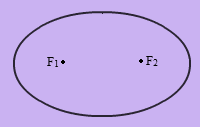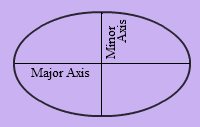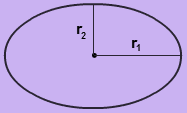# Ellipse Formula

In geometry, an ellipse is described as a curve on a plane that surrounds two focal points such that the sum of the distances to the two focal points is constant for every point on the curve. In the following figure, F1 and F2 are called the foci of the ellipse.Ellipse has two types of axes – Major Axis and Minor Axis. The longest chord of the ellipse is the major axis. The perpendicular chord to the major axis is the minor axis which bisects the major axis at the centre.#### Ellipse Formula

$\large Area\;of\;the\;Ellipse=\pi r_{1}r_{2}$

$\large Perimeter\;of\;the\;Ellipse=2\pi \sqrt{\frac{r_{1}^{2}+r_{2}^{2}}{2}}$

Where,
r1 is the semi major axis of the ellipse.
r2 is the semi minor axis of the ellipse.

### Solved Example

Question: Find the area and perimeter of an ellipse whose semi-major axis is 10 cm and semi-minor axis is 5 cm.
Solution:

Given,
Semi major axis of the ellipse = r1 = 10 cm
Semi minor axis of the ellipse = r2 = 5 cm
Area of the ellipse
= πr1r2
= 3.14
$$\begin{array}{l}\times\end{array}$$
10
$$\begin{array}{l}\times\end{array}$$
5 cm2
= 157 cm2
Perimeter of the ellipse
= 2π
$$\begin{array}{l}\sqrt{\frac{r_{1}^{2}+r_{2}^{2}}{2}}\end{array}$$
= 2π
$$\begin{array}{l}\sqrt{\frac{10^{2}+5^{2}}{2}}\end{array}$$
cm
= 2π
$$\begin{array}{l}\sqrt{\frac{100+25}{2}}\end{array}$$
cm
= 2π
$$\begin{array}{l}\sqrt{\frac{125}{2}}\end{array}$$
cm
= 2 × 3.14 × 7.91 cm
= 49.674 cm
 More topics in Ellipse Formula Volume of an Ellipsoid Formula